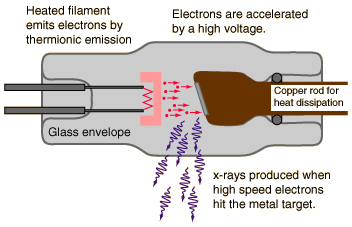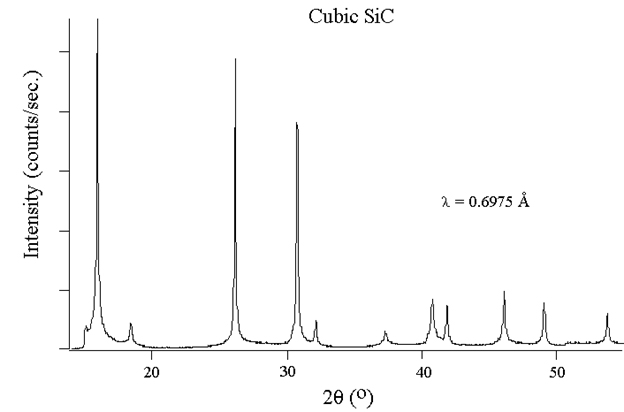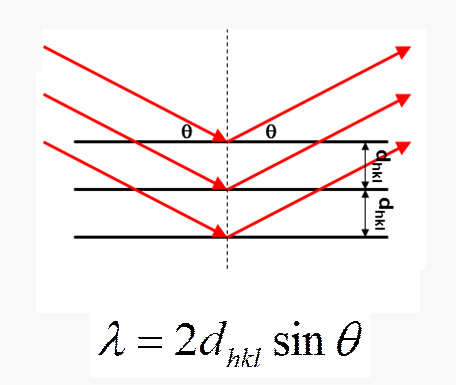Resources
Introduction to X-Ray Diffractometers
1. What are X-Rays?

• Originate in energy shells of atom
• Produced when electrons interact with a target.
2. How X-rays are produced?

• When fast-moving electrons slam into a metal object, x-rays are produced .The kinetic energy of the electron is transformed into electromagnetic energy.3. How X-ray Powder Diffraction Work?

• X-ray diffractometers consist of three basic elements: an X-ray tube, a sample holder, and an X-ray detector.
• When a monochromatic x-ray beam with wavelength l is incident on the lattice planes in a crystal planes in a crystal at an angle q, diffraction occurs only when the distance traveled by the rays reflected from successive planes differs by a complete number n of wavelengths. By varying the angleq, the Bragg’s Law conditions are satisfied by different d-spacing in polycrystalline materials. Plotting the angular positions and intensities of the resultant diffraction peaks produces a pattern which is characterized of the sample. Where a mixture of different phases is present, the diffractogram is formed by addition of the individual patterns.4. Bragg's Law

• For parallel planes of atoms, with a space dhkl between them, constructive interference only occurs when Bragg’s law is satisfied. The X-ray wavelength ƛ is fixed. Each plane of atoms produces a diffraction peak at a specific angle q. The direction perpendicular to the planes must bisect the incident and diffracted beams.5. What can we do with XRD?